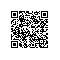# 通过代码审计找出网站中的XSS漏洞实战(三)

1. 找出关键位置
2. 正向审计
3. 反向审计

## 三、找出关键位置

### 3.1 找出控制器

http://permeate.songboy.net/home/index.php

http://permeate.songboy.net/home/index.php?m=tiezi&a=index&bk=6

<?php
require_once "../core/common.php";
include "./public/header.php";
includeAction("$model","$action");
include "./public/footer.php";

function includeAction($model,$action)
{
//判断控制器是否存在
$filePath = "./action/$model.php";
if (is_readable($filePath)) { require_once$filePath;
$class = new$model;
if (is_callable(array($class,$action))) {
$class->$action();
return true;
}
}

//如果没有找到对应的控制器，直接调用模板文件
$tplFilePath = "./tpl/$model/$action.php"; if (is_readable($tplFilePath)) {
require_once $tplFilePath; return true; } echo '控制器或模板文件' .$filePath . '不存在!';
die;
}http://permeate.songboy.net/home/index.php?m=tiezi&a=index&bk=6

<?php

class tiezi
{
function __construct()
{

}

public function index()
{
.....
$data['count'] =$count;
$data['page_size'] =$page_size;
$data['page_count'] =$page_count;
$data['page_num'] =$page_num;
displayTpl('tiezi/index', $data); } 果然发现了index方法 ### 3.2 找出模板 得到控制器之后，笔者还需要找到模板存放的位置，通常模板与控制器是息息相关，因此可以控制其中找到蛛丝马迹，比如上面的代码当中，最后一行代码为displayTpl函数，从字面意思上可以理解为显示模板，因此笔者通过PHPStorm的跳转功能直接跳过去查看该函数的具体流程，找到代码如下所示 /** * 加载模板文件 * @param$tplPath
*/
function displayTpl($tplPath,$data = [])
{
$filePath = "./tpl/$tplPath.php";
if (!is_readable($filePath)) { echo '模板文件' .$filePath . '不存在!';
die;
}

foreach ($data as$key => $val) {$$key =$val;
}

require_once $filePath; } 在上面代码当中可以看出模板存放于home/tpl目录下，通过文件夹打开查看，如下图所示### 3.3 验证位置 通过上面的操作流程已经基本确定控制器与模板的位置，但为了防止意外，还是准确验证一下，在控制器中输出一个字符串1111111，在模板中输出字符串222222222，如果按照笔者之前所预想的，那么这两组字符串都会被输出，参考代码如下 在控制器中加入的测试代码如下 public function index() { echo '11111111111'; 在模板文件中加入的测试代码如下 222222222222222 <?php$get = $_GET; ?> <section class="section"> 现在会到浏览器，在当前页面单击鼠标右键，选中查看源代码，如下图所示在源代码当中，搜索字符串11111,果然搜索到字符串，如下图所示## 四、正向审计 在找到关键位置之后，笔者便可以针对性的去进行代码审计，XSS的代码审计主要有两种方式，正向代码审计，反向代码审计；正向代码审计的意思是从参数的接收到参数最后的使用这个流程进行检查，而反向审计则是相反从变量使用的位置上推到参数接收 ### 4.1 接收参数位置 首先通过正向方式来进行代码审计，正向代码审计是从接收参数进行排查，因此找到控制器当中，通过编辑器的搜索功能，笔者在控制器文件当中搜索了关键字$_GET 找到了tiezi.php控制器中的index方法，代码如下所示

    public function index()
{
$id =$_GET['bk'];
$bk = &$id;

//开始分页大小
$page_size = 15; //获取当前页码$page_num = empty($_GET['page']) ? 1 :$_GET['page'];

//中间代码.................省略

$data['bk'] =$bk;
$data['count'] =$count;
$data['page_size'] =$page_size;
$data['page_count'] =$page_count;
$data['page_num'] =$page_num;
displayTpl('tiezi/index', $data); } ### 4.2 模板位置是否过滤 从上面代码当中可以看出参数bk并没有进行任何过滤，便直接放到了模板当中，这便留下安全隐患，如果在模板当中也没用进行安全过滤，那么就存在着反射型XSS漏洞，打开模板文件并搜索关键词bk，代码如下所示 <div class="post-list-controller"> <div style="float: right"> <a class="btn btn-primary" href="fatie.php?bk=<?php echo$bk ?>">发帖</a>
</div>

### 4.3 漏洞验证

http://permeate.songboy.net/home/index.php?m=tiezi&a=index&bk=6%22%3E%3Cscript%3Ealert(123)%3C/script%3E## 五、反向审计

### 5.1 找出模板中的变量

echo \$([a-z]*) 这个正则表达式是匹配输出变量,比如匹配字符echo$zhangsan，用PHPStorm匹配到的结果如下图所示### 5.2 查找变量来源

$where = " where title like '%$keywords%' ";

### 5.3 漏洞验证

http://permeate.songboy.net/home/search.php?keywords=%E6%B5%8B%E8%AF%95%3Cscript%3Ealert(123)%3C/script%3E## 六、新书推荐使用钉钉扫一扫加入圈子
+ 订阅